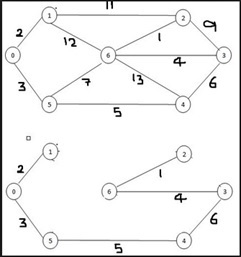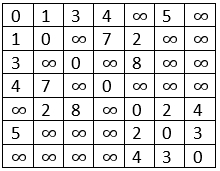# Kruskal’s Minimum Spanning Tree Algorithm

Greedy AlgorithmData StructureAlgorithms

#### Analysis of Algorithm

80 Lectures 7 hours

#### Design and Analysis of an Algorithm

15 Lectures 2.5 hours

#### 100 JavaScript Algorithm Challenges

116 Lectures 7.5 hours

There is a connected graph G(V, E) and the weight or cost for every edge is given. Kruskal’s algorithm will find the minimum spanning tree using the graph and the cost.

It is the merge-tree approach. Initially, there are different trees, this algorithm will merge them by taking those edges whose cost is minimum, and form a single tree.In this problem, all of the edges are listed and sorted based on their cost. From the list, edges with minimum costs are taken out and added in the tree, and every there is a check whether the edge forming cycle or not, if it forms a cycle then discard the edge from the list and go for next edge.

The time complexity of this algorithm is O(E log E) or O(E log V), where E is a number of edges and V is a number of vertices.

## Input and Output

Input:Output:
Edge: B--A And Cost: 1
Edge: E--B And Cost: 2
Edge: F--E And Cost: 2
Edge: C--A And Cost: 3
Edge: G--F And Cost: 3
Edge: D--A And Cost: 4
Total Cost: 15

## Algorithm

kruskal(g: Graph, t: Tree)

Input − The given graph g, and an empty tree t

Output − The tree t with selected edges

Begin
create set for each vertices in graph g
for each set of vertex u do
add u in the vertexSet[u]
done

sort the edge list.
count := 0
while count <= V – 1 do    //as tree must have V – 1 edges
ed := edgeList[count]   //take an edge from edge list
if the starting vertex and ending vertex of ed are in same set then
merge vertexSet[start] and vertexSet[end]
add the ed into tree t
count := count +1
done
End

## Example

#include<iostream>
#define V 7
#define INF 999
using namespace std;

//Cost matrix of the graph
int costMat[V][V] = {
{0, 1, 3, 4, INF, 5, INF},
{1, 0, INF, 7, 2, INF, INF},
{3, INF, 0, INF, 8, INF, INF},
{4, 7, INF, 0, INF, INF, INF},
{INF, 2, 8, INF, 0, 2, 4},
{5, INF, INF, INF, 2, 0, 3},
{INF, INF, INF, INF, 4, 3, 0}
};

typedef struct {
int u, v, cost;
}edge;

void swapping(edge &e1, edge &e2) {
edge temp;
temp = e1;
e1 = e2;
e2 = temp;
}

class Tree {
int n;
edge edges[V-1];    //as a tree has vertex-1 edges
public:
Tree() {
n = 0;
}

void addEdge(edge e) {
edges[n] = e;   //add edge e into the tree
n++;
}

void printEdges() {    //print edge, cost and total cost
int tCost = 0;

for(int i = 0; i<n; i++) {
cout << "Edge: " << char(edges[i].u+'A') << "--" <<char(edges[i].v+'A');
cout << " And Cost: " << edges[i].cost << endl;
tCost += edges[i].cost;
}
cout << "Total Cost: " << tCost << endl;
}
};

class VSet {
int n;
int set[V];    //a set can hold maximum V vertices
public:
VSet() {
n = -1;
}

void addVertex(int vert) {
set[++n] = vert;    //add vertex to the set
}

int deleteVertex() {
return set[n--];
}

friend int findVertex(VSet *vertSetArr, int vert);
friend void merge(VSet &set1, VSet &set2);
};

void merge(VSet &set1, VSet &set2) {
//merge two vertex sets together
while(set2.n >= 0)
}

int findVertex(VSet *vertSetArr, int vert) {
//find the vertex in different vertex sets
for(int i = 0; i<V; i++)
for(int j = 0; j<=vertSetArr[i].n; j++)
if(vert == vertSetArr[i].set[j])
return i;   //node found in i-th vertex set
}

int findEdge(edge *edgeList) {
//find the edges from the cost matrix of Graph and store to edgeList
int count = -1, i, j;
for(i = 0; i<V; i++)
for(j = 0; j<i; j++)
if(costMat[i][j] != INF) {
count++;
//fill edge list for the position 'count'
edgeList[count].u = i; edgeList[count].v = j;
edgeList[count].cost = costMat[i][j];
}
return count+1;
}

void sortEdge(edge *edgeList, int n) {
//sort the edges of graph in ascending order of cost
int flag = 1, i, j;

for(i = 0; i<(n-1) && flag; i++) {   //modified bubble sort is used
flag = 0;
for(j = 0; j<(n-i-1); j++)
if(edgeList[j].cost > edgeList[j+1].cost) {
swapping(edgeList[j], edgeList[j+1]);
flag = 1;
}
}
}

void kruskal(Tree &tr) {
int ecount, maxEdge = V*(V-1)/2;   //max n(n-1)/2 edges can have in a graph
edge edgeList[maxEdge], ed;
int uloc, vloc;
VSet VSetArray[V];
ecount = findEdge(edgeList);

for(int i = 0; i < V; i++)
VSetArray[i].addVertex(i);    //each set contains one element
sortEdge(edgeList, ecount);      //ecount number of edges in the graph
int count = 0;

while(count <= V-1) {
ed = edgeList[count];
uloc = findVertex(VSetArray, ed.u);
vloc = findVertex(VSetArray, ed.v);

if(uloc != vloc) {    //check whether source abd dest is in same set or not
merge(VSetArray[uloc], VSetArray[vloc]);
}
count++;
}
}

int main() {
Tree tr;
kruskal(tr);
tr.printEdges();
}

## Output

Edge: B--A And Cost: 1
Edge: E--B And Cost: 2
Edge: F--E And Cost: 2
Edge: C--A And Cost: 3
Edge: G--F And Cost: 3
Edge: D--A And Cost: 4
Total Cost: 15